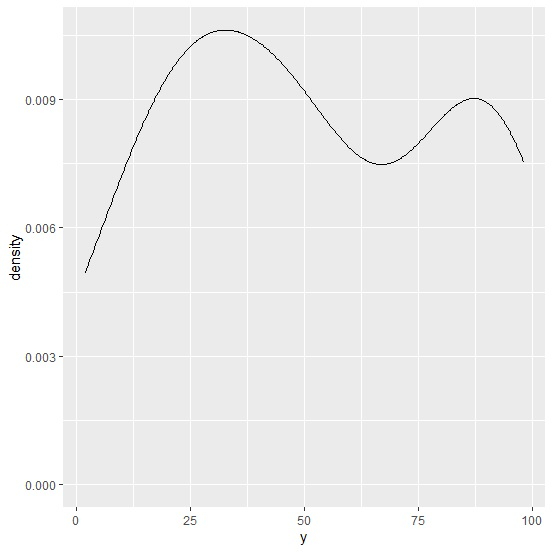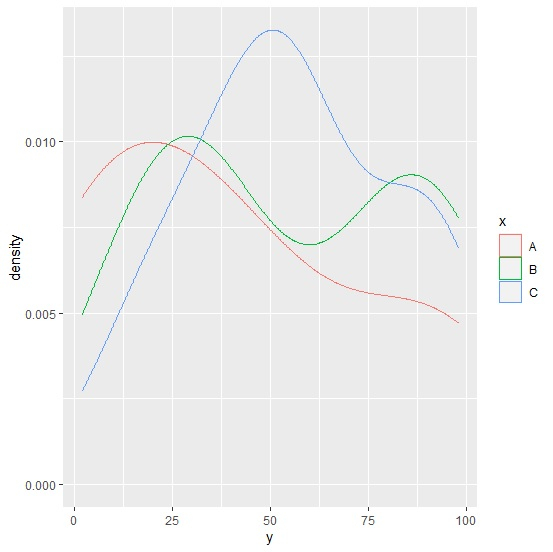# How to create density plot for categories in R?

To create density plot for categories, we can follow the below steps −

• Frist of all, create a data frame.
• Load ggplot2 package and creating the density plot for the whole data.
• Create the density plot for the categories in the data frame by using col function.

## Create the data frame

Let's create a data frame as shown below −

Live Demo

x<-sample(LETTERS[1:3],20,replace=TRUE)
y<-sample(1:100,20)
df<-data.frame(x,y)
df

On executing, the above script generates the below output(this output will vary on your system due to randomization) −

  x y
1 A 47
2 B 46
3 B 29
4 C 53
5 C 60
6 C 17
7 B 79
8 B 12
9 B 30
10 C 91
11 A 92
12 A 2
13 B 25
14 B 98
15 B 88
16 C 34
17 C 50
18 A 20
19 C 90
20 B 87

## Loading ggplot2 package and creating a density plot for whole data

library(ggplot2)
x<-sample(LETTERS[1:3],20,replace=TRUE)
y<-sample(1:100,20)
df<-data.frame(x,y)
ggplot(df,aes(y))+geom_density()

### Output## Create density plot based on categories

Use col function inside aes of geom_density function to create the density plot based on categories −

library(ggplot2)
x<-sample(LETTERS[1:3],20,replace=TRUE)
y<-sample(1:100,20)
df<-data.frame(x,y)
ggplot(df,aes(y))+geom_density(aes(col=x),alpha=0.2)

### Output